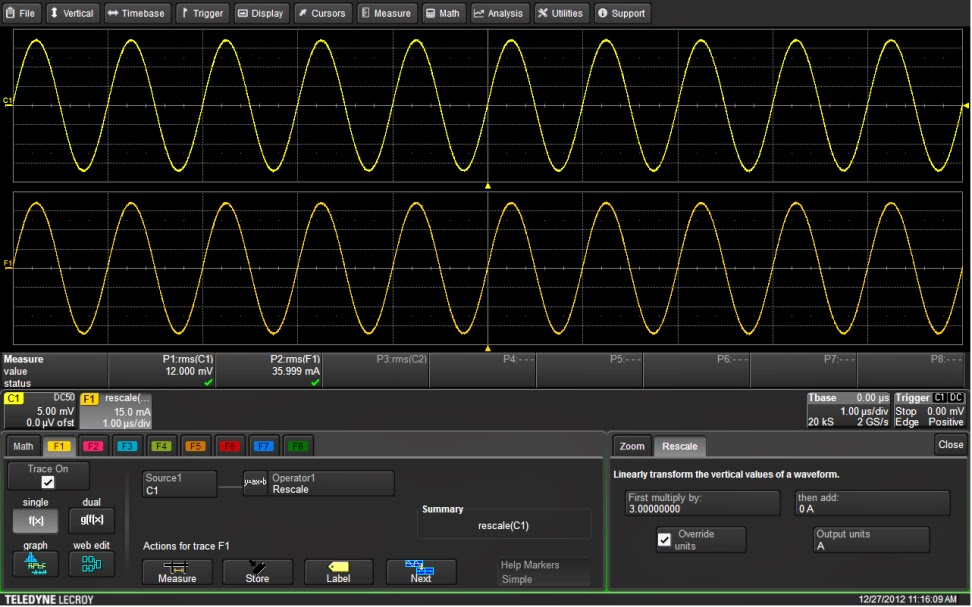## You need to test, we're here to help.

You need to test, we're here to help.

## 19 March 2014

### Tips and Tricks: Rescaling for Non-Voltage MeasurementsFigure 1: Using math rescale to read a current probe's output directly in Amperes
As we know, an oscilloscope is essentially an instrument that measures a constantly varying voltage as a function of time. Those measurements are subsequently analyzed to derive other properties of the input signal, such as frequency, amplitude, rise/fall times, and so on. But what about measurements of other electrical quantities as a function of time?

For example, suppose you're using a Teledyne LeCroy oscilloscope with a third-party current probe, but you still need a readout of the measurement in the proper units (our current probes automatically read in Amperes with no user intervention, but that's another matter). The Rescale function built into Teledyne LeCroy oscilloscopes is what you'll invoke to correct your readout. Figure 1 shows how it would be set up.

The upper trace, Channel 1, is the voltage output of the current probe. According to the probe's manufacturer, the probe's sensitivity is 0.33 mV/mA when operating into a 50-Ω impedance. The dialog box at bottom in Figure 1 summarizes the setup of the rescale function, showing the multiplicative and additive constants as well as the selected units. In this case, we are multiplying the acquired voltage waveform by a factor of 3 to convert from millivolts to milliamps. This is merely the reciprocal of the probe sensitivity: 1/(0.33 mV/mA) = 3 mA/mV.

In addition, the rescale function offers a choice of commonly measured physical units. In this case, Amperes is selected. Once the units are defined, any math operations performed on the rescaled waveform will result in correctly derived units. For example, multiplying voltages by current gives you instantaneous power in Watts. However, for power measurements, the voltage and current waveforms should be deskewed using the instrument's resample function to correctly align them in time.Figure 2: Using the rescale function to convert voltage into SPL in Pascals
Another example of a unit conversion using the rescale function is measuring sound pressure level (SPL) with an instrument microphone as the sensor (Figure 2). In this example, we measured SPL in units of pressure (Pascals or Pa).

The microphone was connected to an SPL calibrator, which generates 110 dB RMS relative to 20 μPa at 1 kHz. In Figure 2, the output of the microphone is in Channel 1. The RMS voltage read by Parameter P4 is 266.21 mV.

First, we convert the calibration level into Pascals. The calibrated 110 dB RMS relative to 20 μPa yields 6.32 Pa. The microphone's sensitivity is 6.32/0.266, or 23.76 Pa/V. This value is entered into the multiplier field in the rescale math function. We checked the Override Units box and the output units are specified as PAL for Pascals. Now, the RMS value of math trace F1 reads 6.32 Pa. Likewise, the FFT of F2 shows an RMS response of 6.32 Pa at 1 kHz.

So these are just a couple of ways to use the rescale function to correctly display non-voltage measurements, complete with correctly scaled and labeled axes. Let us know if you've come up with any others.